Courses

# Ex 4.2 NCERT Solutions- Quadratic Equations Class 10 Notes | EduRev

## Class 10 Mathematics by VP Classes

Created by: Vp Classes

## Class 10 : Ex 4.2 NCERT Solutions- Quadratic Equations Class 10 Notes | EduRev

The document Ex 4.2 NCERT Solutions- Quadratic Equations Class 10 Notes | EduRev is a part of the Class 10 Course Class 10 Mathematics by VP Classes.
All you need of Class 10 at this link: Class 10

Ques 1: Find the roots of the following quadratic equations by factorisation:

(i) x2 − 3x − 10 = 0
(ii) 2x2 + x − 6 = 0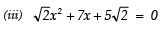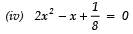Sol: (i) x− 3x − 10 = 0

We have:
x− 3x − 10 = 0
⇒ x2 − 5x + 2x − 10 = 0
⇒ x (x − 5) + 2 (x − 5) = 0
⇒ (x − 5) (x + 2) = 0 Either x − 5=0
⇒ x = 5 or x + 2 = 0
⇒ x = − 2

Thus, the required roots are x = 5 and x = −2.

(ii) 2x2 + x − 6 = 0

We have:

2x2 + x − 6 = 0
⇒ 2x2 + 4x − 3x − 6 =0
⇒ 2x(x + 2) − 3 (x + 2) = 0
⇒ (x + 2) (2x − 3) = 0
Either x + 2 = 0 ⇒ x = −2

or 2x − 3= 0 ⇒ x = 3/2

Thus, the required roots are x = −2 and x = 3/2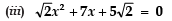We have: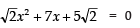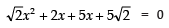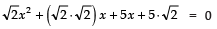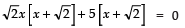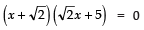Either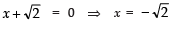or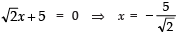Thus, the required roots are x =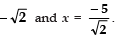(iv)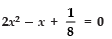We have: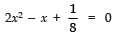⇒ 16x2 − 8x + 1 = 0
⇒ 16x2 − 4x − 4x + 1 = 0
⇒ 4x(4x − 1) − 1(4x − 1) = 0
⇒ (4x − 1) (4x − 1) = 0

⇒ x = 1/4 and x = 1/4

Thus, the required roots are = x = 1/4 and x = 1/4

(v) 100x− 20x + 1 = 0

We have:

100x2 − 20x + 1 = 0
⇒ 100x2 − 10x − 10x + 1 = 0
⇒ 10x (10x − 1) − 1 (10x − 1) = 0
⇒ (10x − 1) (10x − 1) = 0
⇒ (10x − 1) = 0 and (10x − 1) = 0

⇒ x = 1/10 and x = 1/10

Thus, the required roots are x = 1/10 and x = 1/10.

Ques 2: Solve the problems given in Example 1.
Sol: (i) We have: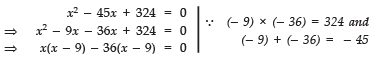⇒ (x − 9) (x − 36) = 0
Either x − 9=0
⇒ x = 9 or x − 36 = 0
⇒ x = 36
Thus, x =9 and x = 36

(ii) We have: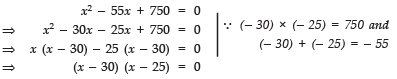Either x − 30 = 0 ⇒ x = 30
or x − 25 = 0 ⇒ x = 25
Thus, x = 30 and x = 25.

Ques 3: Find two numbers whose sum is 27 and product is 182.
Sol: Here, sum of the numbers is 27.
Let one of the numbers be x.
∴ Other number = 27 − x
According to the condition,
Product of the numbers = 182

⇒ x (27 − x) = 182
⇒ 27x − x2 = 182
⇒− x2 + 27x − 182 = 0
⇒ x2 − 27x + 182 = 0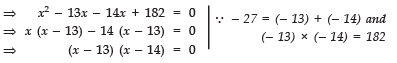Either x − 13 = 0 ⇒ x = 13
or x − 14 = 0 ⇒ x = 14
Thus, the required numbers are 13 and 14.

Ques 4: Find two consecutive positive integers, sum of whose squares is 365.

Sol: Let the two consecutive positive integers be x and (x + 1).

Since the sum of the squares of the numbers = 365

∴ x2 + (x + 1)2 = 365
⇒ x2 + [x2 + 2x + 1] = 365
⇒ x2 + x2 + 2x + 1 = 365
⇒ 2x2 + 2x + 1 − 365 = 0
⇒ 2x2 + 2x − 364 = 0
⇒ x2 + x − 182 = 0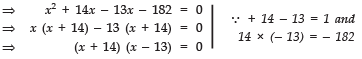Either x + 14 = 0 ⇒ x = − 14
or x − 13 = 0 ⇒ x = 13
Since x has to be a positive integer
∴ x = 13
⇒ x + 1 = 13 + 1 = 14

Thus, the required consecutive positive integers are 13 and 14.

Ques 5: The altitude of a right triangle is 7 cm less than its base. If the hypotenuse is 13 cm, find the other two sides.

Sol: Let the base of the given right triangle be ‘x’ cm.

∴ Its height = (x − 7) cm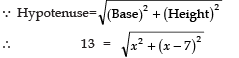Squaring both sides, we get

169 = x2 + (x − 7)2
⇒ 169 = x2 + x2 − 14x + 49
⇒ 2x2 − 14x + 49 − 169 = 0
⇒ 2x2 − 14x − 120 = 0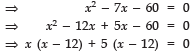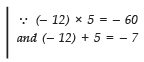⇒ (x − 12) (x + 5) = 0
Either x − 12 = 0  ⇒ x = 12
or x + 5 = 0  ⇒ x = − 5

But the side of triangle can never be negative,

⇒ x = 12
∴ Length of the base = 12 cm
⇒ Length of the height = (12 − 7) cm = 5 cm

Thus, the required base = 12 cm and height = 5 cm.

Ques 6: A cottage industry produces a certain number of pottery articles in a day. It was observed on a particular day that the cost of production of each article (in rupees) was 3 more than twice the number of articles produced on that day. If the total cost of production on that day was Rs 90, find the number of articles produced and the cost of each article.

Sol: Let the number of articles produced in a day = x
∴ Cost of production of each article = ₹ (2x + 3)
According to the condition,

Total cost = 90
⇒ x × (2x + 3) = 90
⇒ 2x2 + 3x = 90
⇒ 2x2 + 3x − 90 = 0
⇒ 2x2 − 12x + 15x − 90 = 0
⇒ 2x (x − 6) + 15 (x − 6) = 0
⇒ (x − 6) (2x + 15) = 0

Either x − 6 = 0 ⇒ x = 6

or 2x + 15 = 0 ⇒ x = -15/2

But the number of articles cannot be negative.

x = -15/2 is not required

⇒ x = 6

∴ Cost of each article = ₹ (2 × 6 + 3) = ₹ 15
Thus, the required number of articles produced is 6 and the cost of each article is ₹15.

Offer running on EduRev: Apply code STAYHOME200 to get INR 200 off on our premium plan EduRev Infinity!

132 docs

,

,

,

,

,

,

,

,

,

,

,

,

,

,

,

,

,

,

,

,

,

;